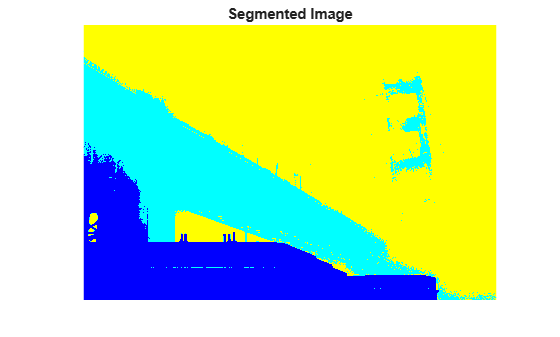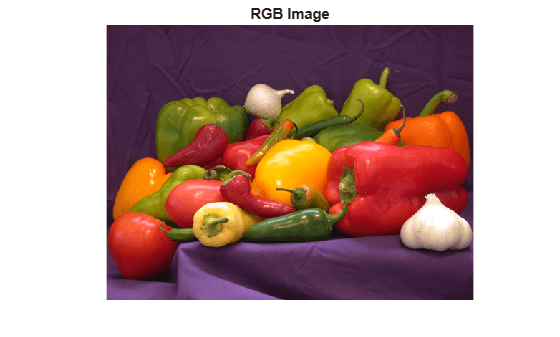# imquantize

Quantize image using specified quantization levels and output values

## Syntax

``quant_A = imquantize(A,levels)``
``quant_A = imquantize(___,values)``
``````[quant_A,index] = imquantize(___)``````

## Description

example

````quant_A = imquantize(A,levels)` quantizes image `A` using specified quantization values contained in the `N` element vector `levels`. Output image `quant_A` is the same size as `A` and contains `N + 1` discrete integer values in the range `1` to `N + 1` which are determined by the following criteria:If A(k) ≤ levels(1), then quant_A(k) = 1.If levels(m-1) < A(k)  ≤  levels(m) , then quant_A(k) = m.If A(k) > levels(N), then quant_A(k) = N + 1.Note that `imquantize` assigns values to the two implicitly defined end intervals:A(k) ≤ levels(1)A(k) > levels(N)```

example

````quant_A = imquantize(___,values)` adds the `N + 1` element vector `values` where `N` = `length`(`levels`). Each of the `N + 1` elements of `values` specify the quantization value for one of the `N + 1` discrete pixel values in `quant_A`.If A(k) ≤ levels(1), then quant_A(k) = values(1).If levels(m-1) < A(k)  ≤  levels(m) , then quant_A(k) = values(m).If A(k) > levels(N), then quant_A(k) = values(N + 1).```

example

``````[quant_A,index] = imquantize(___)``` returns an array index such that:`quant_A` = `values(index)````

## Examples

collapse all

Read an image, convert it to grayscale, and display the result.

```I = imread("foggysf2.jpg"); I = rgb2gray(I); imshow(I) title("Grayscale Image")```Calculate two threshold levels.

`thresh = multithresh(I,2);`

Segment the image into three levels using `imquantize` .

`labels = imquantize(I,thresh);`

Convert the segmented image into a color image using `label2rgb` and display it.

```labelsRGB = label2rgb(labels); imshow(labelsRGB) title("Segmented Image")```Read truecolor (RGB) image and display it.

```I = imread('peppers.png'); imshow(I) axis off title('RGB Image');```Generate thresholds for seven levels from the entire RGB image.

`threshRGB = multithresh(I,7);`

Generate thresholds for each plane of the RGB image.

```threshForPlanes = zeros(3,7); for i = 1:3 threshForPlanes(i,:) = multithresh(I(:,:,i),7); end```

Process the entire image with the set of threshold values computed from entire image.

```value = [0 threshRGB(2:end) 255]; quantRGB = imquantize(I, threshRGB, value);```

Process each RGB plane separately using the threshold vector computed from the given plane. Quantize each RGB plane using threshold vector generated for that plane.

```quantPlane = zeros( size(I) ); for i = 1:3 value = [0 threshForPlanes(i,2:end) 255]; quantPlane(:,:,i) = imquantize(I(:,:,i),threshForPlanes(i,:),value); end quantPlane = uint8(quantPlane);```

Display both posterized images and note the visual differences in the two thresholding schemes.

```imshowpair(quantRGB,quantPlane,'montage') axis off title('Full RGB Image Quantization Plane-by-Plane Quantization')```To compare the results, calculate the number of unique RGB pixel vectors in each output image. Note that the plane-by-plane thresholding scheme yields about 23% more colors than the full RGB image scheme.

```dim = size( quantRGB ); quantRGBmx3 = reshape(quantRGB, prod(dim(1:2)), 3); quantPlanemx3 = reshape(quantPlane, prod(dim(1:2)), 3); colorsRGB = unique(quantRGBmx3, 'rows' ); colorsPlane = unique(quantPlanemx3, 'rows' ); disp(['Unique colors in RGB image : ' int2str(length(colorsRGB))]);```
```Unique colors in RGB image : 188 ```
`disp(['Unique colors in Plane-by-Plane image : ' int2str(length(colorsPlane))]);`
```Unique colors in Plane-by-Plane image : 231 ```

Reduce the number of discrete levels in an image from 256 to 8. This example uses two different methods for assigning values to each of the eight output levels.

```I = imread('coins.png'); imshow(I) axis off title('Grayscale Image')```Split the image into eight levels by obtaining seven thresholds from the `multithresh` function.

`thresh = multithresh(I,7);`

Construct the `valuesMax` vector such that the maximum value in each quantization interval is assigned to the eight levels of the output image.

`valuesMax = [thresh max(I(:))]`
```valuesMax = 1x8 uint8 row vector 65 88 119 149 169 189 215 255 ```
`[quant8_I_max, index] = imquantize(I,thresh,valuesMax);`

Similarly, construct the `valuesMin` vector such that the minimum value in each quantization interval is assigned to the eight levels of the output image. Instead of calling `imquantize` again with the vector `valuesMin`, use the output argument index to assign those values to the output image.

`valuesMin = [min(I(:)) thresh]`
```valuesMin = 1x8 uint8 row vector 23 65 88 119 149 169 189 215 ```
`quant8_I_min = valuesMin(index);`

Display both eight-level output images side by side.

```imshowpair(quant8_I_min,quant8_I_max,'montage') title('Minimum Interval Value Maximum Interval Value')```## Input Arguments

collapse all

Input image, specified as a numeric array of any dimension.

Data Types: `single` | `double` | `int8` | `int16` | `int32` | `int64` | `uint8` | `uint16` | `uint32` | `uint64`

Quantization levels, specified as an `N` element vector. Values of the discrete quantization levels must be in monotonically increasing order.

Data Types: `single` | `double` | `int8` | `int16` | `int32` | `int64` | `uint8` | `uint16` | `uint32` | `uint64`

Quantization values, specified as an `N+1` element vector.

Data Types: `single` | `double` | `int8` | `int16` | `int32` | `int64` | `uint8` | `uint16` | `uint32` | `uint64`

## Output Arguments

collapse all

Quantized output image, returned as a numeric array the same size as A. If input argument values is specified, then quant_A is the same data type as values. If values is not specified, then quant_A is of class `double`.

Mapping array, returned as an array the same size as input image A. It contains integer indices which access values to construct the output image: `quant_A` = `values`(`index`). If input argument values is not defined, then `index` = `quant_A`.

Data Types: `double`

## Version History

Introduced in R2012b

expand all